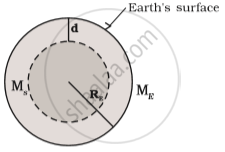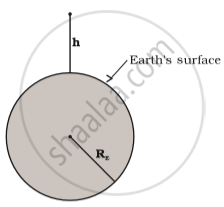Department of Pre-University Education, KarnatakaPUC Karnataka Science Class 11

# Acceleration Due to Gravity Below and Above the Earth's Surface

#### notes

Acceleration due to gravity below the surface of earth

• To calculate acceleration due to gravity below the surface of the earth (between the surface and centre of the earth).

Density of the earth is constant throughout. Therefore,

ρ = M/ (4/3π Re3)          ....(1)

where,

M= mass of the earth

Volume of sphere= 4/3π "R"_e^2

Re = radius of the earth.

As entire mass is concentrated at the centre of the earth.

Therefore density can be written as

rho = M_s(4/3π "R"_s^3)      ....(2)

Comparing equation (1) and (2)

"M"_e/"M"_s="R"_e^3/"R"_s^3      "where"  "R"_s= ("R"_e-"d")^3

d= distance of the body form the centre to the surface of the earth.

Therefore,

"M"_e/"M"_s="R"_e^3/("R"_e-"d")^3

"M"_s = "M"_e(("R"_e-"d")^3)/"R"_e^3        ...(3)

To calculate Gravitational force (F) between earth and point mass m at a depth d below the surface of the earth.Above figure shows the value of g at a depth d. In this case only the smaller sphere of radius ("R"_e- "d") contributes to g.

"F" ="GmM"_s/("R"_e-"d")^2

"g"="F"/"m"  where g=acceleration due to gravity at point 'd' below the surface of the earth.

"g"="GM"_s/("R"_e-"d")^2

Putting the value of "M"_s from Eq.(3)

="GM"_e("R"_e-"d")^3/("R"_e^3("R"_e-"d")^2)

="GM"_e(R_e-d)/R_e^3

W.k.t.    "g"="GM"_e/"R"_e^2

g(d)="GM"_e/R_e^3("R"_e-"d")

=g((R_e-d)/R_e)=g(1-d/R_e)

Acceleration due to gravity above the surface of the earth:

• To calculate the value of acceleration due to gravity of a point mass m at a height h above the surface of the earth.

• Force of gravitation between the object and the earth will beF(h)=("GM"_Em)/(R_E + h)^2

The acceleration experienced by the point mass is ("F"("h"))/"m"≡"g"("h")

"g"(h)=("F"(h))/m="GM"_E/("R"_E + h)^2 ...(1)

This is cleary less than the value of g on the surface of earth: "g"="GM"_E/"R"_E^2 for h<<RE, we can expand the RHS of Eq.(1)

g(h)="GM"_E/(R_E^2 (1+h/R_E)^2)

=g(1+h/"R"_E)^-2

For "h"/"R"_E<<1. Using binomial expression,

"g"(h)cong"g"(1-(2h)/R_E)

Conclusion: The value of acceleration due to gravity varies on the surface, above surface and below the surface of the earth.

#### description

• Variation of g with altitude
• Variation of g with depth
• Graph of g, R and d
If you would like to contribute notes or other learning material, please submit them using the button below.